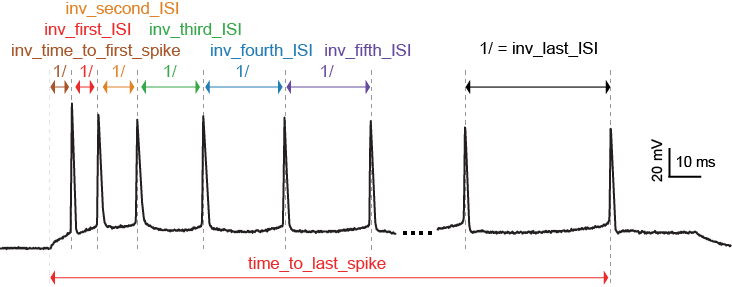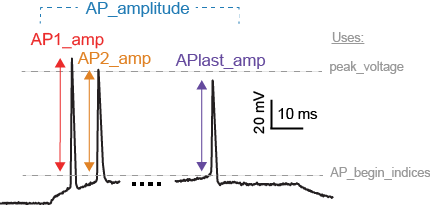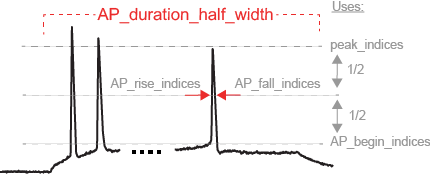# eFeature descriptions¶

A pdf document describing the eFeatures is available here.

Not every eFeature has a description in this document yet, the complete set will be available shortly.

## Implemented eFeatures (to be continued)¶

### Spike event features¶LibV5 : inv_time_to_first_spike

1.0 over time to first spike; returns 0 when no spike

• Required features: time_to_first_spike

• Units: Hz

• Pseudocode:

```if len(time_to_first_spike) > 0:
inv_time_to_first_spike = 1.0 / time_to_first_spike
else:
inv_time_to_first_spike = 0
```

LibV5 : inv_first_ISI, inv_second_ISI, inv_third_ISI, inv_fourth_ISI, inv_fith_ISI, inv_last_ISI

1.0 over first/second/third/fourth/fith/last ISI; returns 0 when no ISI

• Required features: peak_time (ms)

• Units: Hz

• Pseudocode:

```all_isi_values_vec = numpy.diff(peak_time)
if len(all_isi_values_vec) > 1:
inv_first_ISI = 1000.0 / all_isi_values_vec
else:
inv_first_ISI = 0

if len(all_isi_values_vec) > 0:
inv_first_ISI = 1000.0 / all_isi_values_vec
else:
inv_first_ISI = 0

if len(all_isi_values_vec) > 1:
inv_second_ISI = 1000.0 / all_isi_values_vec
else:
inv_second_ISI = 0

if len(all_isi_values_vec) > 2:
inv_third_ISI = 1000.0 / all_isi_values_vec
else:
inv_third_ISI = 0

if len(all_isi_values_vec) > 3:
inv_fourth_ISI = 1000.0 / all_isi_values_vec
else:
inv_fourth_ISI = 0

if len(all_isi_values_vec) > 4:
inv_fifth_ISI = 1000.0 / all_isi_values_vec
else:
inv_fifth_ISI = 0

if len(all_isi_values_vec) > 0:
inv_last_ISI = 1000.0 / all_isi_values_vec[-1]
else:
inv_last_ISI = 0
```

LibV5 : time_to_last_spike

time from stimulus start to last spike

• Required features: peak_time (ms), stimstart (ms)

• Units: ms

• Pseudocode:

```if len(peak_time) > 0:
time_to_last_spike = peak_time[-1] - stimstart
else:
time_to_last_spike = 0
```

LibV1 : Spikecount

number of spikes in the trace, including outside of stimulus interval

• Required features: LibV1:peak_indices

• Units: constant

• Pseudocode:

```Spikecount = len(peak_indices)
```

LibV5 : Spikecount_stimint

number of spikes inside the stimulus interval

• Required features: LibV1:peak_time

• Units: constant

• Pseudocode:

```peaktimes_stimint = numpy.where((peak_time >= stim_start) & (peak_time <= stim_end))
Spikecount_stimint = len(peaktimes_stimint)
```

LibV5 : number_initial_spikes

number of spikes at the beginning of the stimulus

• Required features: LibV1:peak_time

• Required parameters: initial_perc (default=0.1)

• Units: constant

• Pseudocode:

```initial_length = (stimend - stimstart) * initial_perc
number_initial_spikes = len(numpy.where( \
(peak_time >= stimstart) & \
(peak_time <= stimstart + initial_length)))
```

LibV5 : ISI_semilog_slope

The slope of a linear fit to a semilog plot of the ISI values

• Required features: t, V, stim_start, stim_end, ISI_values

• Units: ms

• Pseudocode:

```x = range(1, len(ISI_values)+1)
log_ISI_values = numpy.log(ISI_values)
slope, _ = numpy.polyfit(x, log_ISI_values, 1)

ISI_semilog_slope = slope
```

LibV5 : ISI_log_slope

The slope of a linear fit to a loglog plot of the ISI values

• Required features: t, V, stim_start, stim_end, ISI_values

• Units: ms

• Pseudocode:

```log_x = numpy.log(range(1, len(ISI_values)+1))
log_ISI_values = numpy.log(ISI_values)
slope, _ = numpy.polyfit(log_x, log_ISI_values, 1)

ISI_log_slope = slope
```

### Spike shape features¶LibV1 : AP_Amplitude, AP1_amp, AP2_amp, APlast_amp

The relative height of the action potential from spike onset

• Required features: LibV5:AP_begin_indices, LibV1:peak_voltage (mV)

• Units: mV

• Pseudocode:

```AP_Amplitude = voltage[AP_begin_indices] - peak_voltage
AP1_amp = AP_Amplitude
AP2_amp = AP_Amplitude
APlast_amp = AP_Amplitude[-1]
```LibV1 : AHP_depth_abs

Absolute voltage values at the first after-hyperpolarization

• Required features: LibV5:min_AHP_values (mV)
• Units: mV

LibV1 : AHP_depth_abs_slow

Absolute voltage values at the first after-hyperpolarization starting 5 ms after the peak

• Required features: LibV1:peak_indices
• Units: mV

LibV1 : AHP_slow_time

Time difference between slow AHP (see AHP_depth_abs_slow) and peak, divided by interspike interval

• Required features: LibV1:AHP_depth_abs_slow
• Units: constant

LibV1 : AHP_depth

Relative voltage values at the first after-hyperpolarization

• Required features: LibV1:voltage_base (mV), LibV5:min_AHP_values (mV)

• Units: mV

• Pseudocode:

```min_AHP_values = first_min_element(voltage, peak_indices)
AHP_depth = min_AHP_values[:] - voltage_base
```

LibV5 : AHP_time_from_peak

Time between AP peaks and first AHP depths

• Required features: LibV1:peak_indices, LibV5:min_AHP_values (mV)

• Units: mV

• Pseudocode:

```min_AHP_indices = first_min_element(voltage, peak_indices)
AHP_time_from_peak = t[min_AHP_indices[:]] - t[peak_indices[i]]
```LibV2 : AP_duration_half_width

Width of spike at half spike amplitude

• Required features: LibV2: AP_rise_indices, LibV2: AP_fall_indices

• Units: ms

• Pseudocode:

```AP_rise_indices = index_before_peak((v(peak_indices) - v(AP_begin_indices)) / 2)
AP_fall_indices = index_after_peak((v(peak_indices) - v(AP_begin_indices)) / 2)
AP_duration_half_width = t(AP_fall_indices) - t(AP_rise_indices)
```

LibV1 : AP_width

Width of spike at threshold

• Required features: LibV1: peak_indices, LibV5: min_AHP_indices, threshold

• Units: ms

• Pseudocode:

```min_AHP_indices.append(stim_start_index)
for i in range(len(min_AHP_indices)-1):
onset_time[i] = t[numpy.where(v[min_AHP_indices[i]:min_AHP_indices[i+1]] > threshold)]
offset_time[i] = t[numpy.where(v[min_AHP_indices[i]:min_AHP_indices[i+1]] < threshold && t > onset_time)]
AP_width[i] = t(offset_time[i]) - t(onset_time[i])
```

### Voltage features¶The average voltage during the last 10% of the stimulus duration.

• Required features: t, V, stim_start, stim_end

• Units: mV

• Pseudocode:

```stim_duration = stim_end - stim_start
begin_time = stim_end - 0.1 * stim_duration
end_time = stim_end
steady_state_voltage_stimend = numpy.mean(voltage[numpy.where((t < end_time) & (t >= begin_time))])
```

The average voltage after the stimulus

• Required features: t, V, stim_end

• Units: mV

• Pseudocode:

```steady_state_voltage = numpy.mean(voltage[numpy.where((t <= max(t)) & (t > stim_end))])
```

#### LibV5 : voltage_base¶

The average voltage during the last 10% of time before the stimulus.

• Required features: t, V, stim_start, stim_end

• Parameters: voltage_base_start_perc (default = 0.9)

voltage_base_end_perc (default = 1.0)

• Units: mV

• Pseudocode:

```voltage_base = numpy.mean(voltage[numpy.where(
(t >= voltage_base_start_perc * stim_start) &
(t <= voltage_base_end_perc * stim_start))])
```

#### LibV5 : decay_time_constant_after_stim¶

The decay time constant of the voltage right after the stimulus

• Required features: t, V, stim_start, stim_end

• Parameters: decay_start_after_stim (default = 1.0 ms)

decay_end_after_stim (default = 10.0 ms)

• Units: ms

• Pseudocode:

```time_interval = t[numpy.where(t => decay_start_after_stim &
t < decay_end_after_stim)] - t[numpy.where(t == stim_end)]
voltage_interval = abs(voltages[numpy.where(t => decay_start_after_stim &
t < decay_end_after_stim)]
- voltages[numpy.where(t == decay_start_after_stim)])

log_voltage_interval = numpy.log(voltage_interval)
slope, _ = numpy.polyfit(time_interval, log_voltage_interval, 1)

decay_time_constant_after_stim = -1. / slope
```#### LibV5 : sag_amplitude¶

The difference between the minimal voltage and the steady state at stimend

• Required features: t, V, stim_start, stim_end, steady_state_voltage_stimend, minimum_voltage, voltage_deflection_stim_ssse

• Parameters:

• Units: mV

• Pseudocode:

```if (voltage_deflection_stim_ssse <= 0):
else:
sag_amplitude = None
```

#### LibV5 : sag_ratio1¶

The ratio between the sag amplitude and the maximal sag extend from voltage base

• Required features: t, V, stim_start, stim_end, sag_amplitude, voltage_base, minimum_voltage

• Parameters:

• Units: constant

• Pseudocode:

```if voltage_base != minimum_voltage:
sag_ratio1 = sag_amplitude / (voltage_base - minimum_voltage)
else:
sag_ratio1 = None
```

#### LibV5 : sag_ratio2¶

The ratio between the maximal extends of sag from steady state and voltage base

• Required features: t, V, stim_start, stim_end, steady_state_voltage_stimend, voltage_base, minimum_voltage

• Parameters:

• Units: constant

• Pseudocode:

```if voltage_base != minimum_voltage:
sag_ratio2 = (voltage_base - steady_state_voltage_stimend) / (voltage_base - minimum_voltage)
else:
sag_ratio2 = None
```

#### LibV1 : ohmic_input_resistance¶

The ratio between the voltage deflection and stimulus current

• Required features: t, V, stim_start, stim_end, voltage_deflection

• Parameters: stimulus_current

• Units: mV/nA

• Pseudocode:

```ohmic_input_resistance = voltage_deflection / stimulus_current
```

#### LibV5 : ohmic_input_resistance_vb_ssse¶

The ratio between the voltage deflection (between voltage base and steady-state voltage at stimend) and stimulus current

• Required features: t, V, stim_start, stim_end, voltage_deflection_vb_ssse

• Parameters: stimulus_current

• Units: mV/nA

• Pseudocode:

```ohmic_input_resistance_vb_ssse = voltage_deflection_vb_ssse / stimulus_current
```

#### LibV5 : voltage_deflection_vb_ssse¶

The voltage deflection between voltage base and steady-state voltage at stimend

• Required features: t, V, stim_start, stim_end, voltage_base, steady_state_voltage_stimend

• Units: mV

• Pseudocode:

```voltage_deflection_vb_ssse = steady_state_voltage_stimend - voltage_base
```

## Requested eFeatures¶

LibV1 : AHP_depth_last

Relative voltage values at the last after-hyperpolarization

• Required features: LibV1:voltage_base (mV), LibV5:last_AHP_values (mV)

• Units: mV

• Pseudocode:

```last_AHP_values = last_min_element(voltage, peak_indices)
AHP_depth = last_AHP_values[:] - voltage_base
```

LibV5 : AHP_time_from_peak_last

Time between AP peaks and last AHP depths

• Required features: LibV1:peak_indices, LibV5:min_AHP_values (mV)

• Units: mV

• Pseudocode:

```last_AHP_indices = last_min_element(voltage, peak_indices)
AHP_time_from_peak_last = t[last_AHP_indices[:]] - t[peak_indices[i]]
```

The average voltage during the last 90% of the stimulus duration realtive to voltage_base

• Required features: LibV5: steady_state_voltage_stimend (mV), LibV5: voltage_base (mV)

• Units: mV

• Pseudocode:

```steady_state_voltage_stimend_from_voltage_base = steady_state_voltage_stimend - voltage_base
```

LibV5 : min_duringstim The minimum voltage during stimulus

• Required features:

• Units: mV

• Pseudocode:

```min_duringstim = [numpy.min(voltage[numpy.where((t <= stim_end) & (t >= stim_start))])]
```

LibV5 : min_duringstim_from_voltage_base The minimum voltage during stimulus

• Required features: LibV5: min_duringstim (mV), LibV5: voltage_base (mV)

• Units: mV

• Pseudocode:

```min_duringstim_from_voltage_base = min_duringstim - voltage_base
```

LibV5 : max_duringstim The minimum voltage during stimulus

• Required features:

• Units: mV

• Pseudocode:

```min_duringstim = [numpy.max(voltage[numpy.where((t <= stim_end) & (t >= stim_start))])]
```

LibV5 : max_duringstim_from_voltage_base The minimum voltage during stimulus

• Required features: LibV5: max_duringstim (mV), LibV5: voltage_base (mV)

• Units: mV

• Pseudocode:

```max_duringstim_from_voltage_base = max_duringstim - voltage_base
```

LibV5 : diff_max_duringstim Difference between maximum and steady state during stimulation

• Required features: LibV5: max_duringstim (mV), LibV5: steady_state_voltage_stimend (mV)

• Units: mV

• Pseudocode:

```diff_max_duringstim: max_duringstim - steady_state_voltage_stimend
```

LibV5 : diff_min_duringstim Difference between minimum and steady state during stimulation

• Required features: LibV5: min_duringstim (mV), LibV5: steady_state_voltage_stimend (mV)

• Units: mV

• Pseudocode:

```diff_min_duringstim: min_duringstim - steady_state_voltage_stimend
```